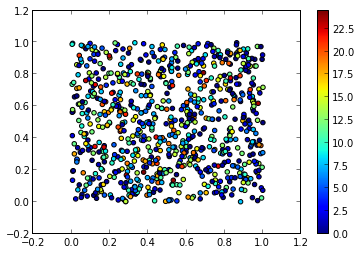## Creation of a 2D balanced network with pyNN¶

### Defining parameters¶

Here we should start by loading the correct pynn backend. Here I'm using nest, but the choice is yours. This could be brian, neuron, nemo, pcsim (experimental)

In :
from pyNN.nest import *


Now we can define the cell parameters. Note that in this example, we will use Integrate and Fire neurons with Current Based synapses. Thus, for stimulating the network, we could either bombard them with Poisson sources, either inject a constant current, or, as we are doing here, select a leak potential above the spike threshold. This is equivalent to current injection.

In :
### Cell parameters ###
tau_m   = 20. # ms Membrane time constant
c_m     = 0.2 # nF Capacitance
tau_exc =  3. # ms Synaptic time constant (excitatory)
tau_inh =  7. # ms Synaptic time constant (inhibitory)
tau_ref =  5. # ms Refractory period
El      = -49 # mV Leak potential
Vt      = -50 # mV Spike Threhold
Vr      = -60 # mV Spike Reset


We can then create the dictionary of those parameters

In :
cell_params = {
'tau_m'      : tau_m, 'tau_syn_E'  : tau_exc, 'tau_syn_I'  : tau_inh,
'v_rest'     : El,    'v_reset'    : Vr,      'v_thresh'   : Vt,
'cm'         : c_m,   'tau_refrac' : tau_ref}


And again, define some more parameters of our simulations

In :
celltype      = IF_curr_exp             # The pyNN celltype we are using
n_cells       = 1000                    # Total number of cells
n_exc         = int(0.8 * n_cells)      # 4:1 ratio for exc/inh
n_inh         = n_cells - n_exc
size          = 1.                      # [arbitrary units] Size of the network
simtime       = 10000                   # [ms] Simulation time
epsilon       = 0.2                     # Probability density for the gaussian connections
s_lat         = 0.15                    # [unit] Spread of the lateral connections
g_exc         = 0.015                   # [nS] Excitatory conductance
g_inh         = -0.15                   # [nS] Inhibitory conductance
velocity      = 0.3                     # [mm/ms]  velocity of axonal delays
dt            = 0.1                     # [ms] timestep of the simulation
max_distance  = size/numpy.sqrt(2)      # [unit] Since this is a torus
max_delay     = dt + max_distance/velocity # [ms] Needed for the connectors
min_delay     = dt                      # [ms]
rngseed       = 92847459                # Random seed
parallel_safe = False                   # To have results independant on number of nodes


Now we can start to setup the simulator, and create the network

In :
node_id = setup(timestep=dt, min_delay=min_delay, max_delay=max_delay+dt)


Once the simulator has been initialized, we can create the populations, and attribute random position to all the cells onto a 2D grid

In :
all_cells              = Population(n_exc+n_inh, celltype, cell_params, label="All Cells")
numpy.random.seed(12)
all_cells.positions    = size*numpy.random.rand(3, n_cells)
all_cells.positions = 0
exc_cells              = all_cells[0:n_exc]
inh_cells              = all_cells[n_exc:n_cells]


Then we initialize the voltages uniformly in the interval [El, Vt]

In :
rng = NumpyRNG(seed=rngseed, parallel_safe=parallel_safe)
uniformDistr = RandomDistribution('uniform', [Vr, 1.1*Vt], rng=rng)
all_cells.initialize('v', uniformDistr)


### Establishing and checking connections¶

This is the nice part: pyNN uses the advantages of python as an interpreted language to provide any rational expression to establish connections. In our case, we want to have a gaussian kernel for lateral connections, and linear delays. Thus, we just have to define

In :
probas   = "%g * exp(-d**2/(2*%g**2))" %(epsilon, s_lat)
delays   = "%g + d/%g" %(dt, velocity)
space    = Space(periodic_boundaries=((0, 1), (0, 1), (0, 1))) #This is a torus, so we are wrapping at edges

exc_conn = DistanceDependentProbabilityConnector(probas, weights=g_exc, delays=delays, space=space)
inh_conn = DistanceDependentProbabilityConnector(probas, weights=g_inh, delays=delays, space=space)

connections        = {}
connections['exc'] = Projection(exc_cells, all_cells, exc_conn, target='excitatory', rng=rng)
connections['inh'] = Projection(inh_cells, all_cells, inh_conn, target='inhibitory', rng=rng)


Here the connections have been created, and the two population are connected with a local profile for the lateral connections, and linear delays. To check that this is indeed the case, we could plot the connections, reading them back from the networks. To do so, let's define functions to calculate distances (on a torus), and to draw what we will call a lateral field, i.e all the incoming/outcoming connections from a cell in the network.

In :
def draw_rf(cell, positions, connections, color='k'):
idx     = numpy.where(connections[:,1] == cell)
sources = connections[idx, 0]
for src in sources:
plot([positions[cell, 1], positions[src, 1]], [positions[cell, 2], positions[src, 2]], c=color)

def distances(pos_1, pos_2, N):
dx = abs(pos_1[:,0]-pos_2[:,0])
dy = abs(pos_1[:,1]-pos_2[:,1])
dx = numpy.minimum(dx, N-dx)
dy = numpy.minimum(dy, N-dy)
return sqrt(dx*dx + dy*dy)


Now, let's ask the network to save its connections, and the positions of the cells, and let's reload them to do some plots with them. We should be able to see that connections are indeed local and delays linear.

In :
connections['exc'].saveConnections('connections.dat')
all_cells.save_positions('positions.dat')


Then, we can compute off line the distances between all those cells, for established connections:

In :
idx_pre          = conn[:,0].astype(int)
idx_post         = conn[:,1].astype(int)
d                = distances(positions[idx_pre,1:3], positions[idx_post,1:3], 1)


Let's see where are all the cell located. Here, we are plotting positions of all exc/inh cells, without sorting them

In :
plot(positions[:,1], positions[:,2], '.')
title('Cells positions')

Out:
<matplotlib.text.Text at 0x7856a50>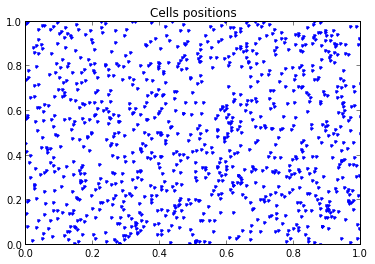We can have a look to the distributions of the delays that have been established, read from the simulator's memory:

In :
d=hist(conn[:,3], 50)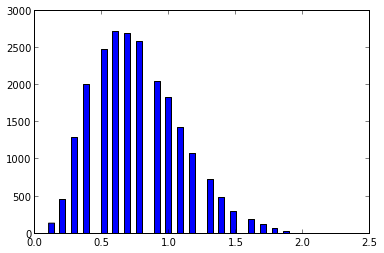And finally, we can plot some lateral field for outgoing connections, to indeed be sure of the established profile for the connections:

In :
ids   = numpy.random.permutation(positions[:,0])[0:6]
colors = ['k', 'r', 'b', 'g', 'c', 'y']
for count, cell in enumerate(ids):
draw_rf(cell, positions, conn, colors[count])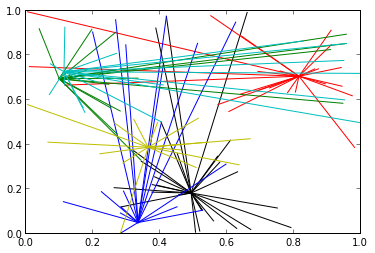### Recording and launching the simulation¶

We are recording the spikes of all the excitatory cells

In :
exc_cells.record(to_file=False)


And then we are running the network and printing spikes. As simple as that:

In :
run(simtime)
exc_cells.printSpikes("spikes.dat")


### Analyzing and plotting spikes and firing rates with NeuroTools¶

Now, if we want to load and play with the spikes, we can use the NeuroTools.signals package, that we need to import:

In :
from NeuroTools.signals import *

/home/pierre/lib/python2.7/site-packages/NeuroTools/__init__.py:114: DependencyWarning: ** TableIO ** package is not installed.
To have functions using TableIO please install the package.
website : http://kochanski.org/gpk/misc/TableIO.html

warnings.warn(get_import_warning(name), DependencyWarning)
/home/pierre/lib/python2.7/site-packages/NeuroTools/__init__.py:114: DependencyWarning: ** interval ** package is not installed.
To have functions using interval please install the package.
website : http://pypi.python.org/pypi/interval/1.0.0

warnings.warn(get_import_warning(name), DependencyWarning)


And we can load spikes, display raster plots and various other funky statistics:

In :
spikes = load_spikelist('spikes.dat', id_list=xrange(800))

In :
spikes.raster_plot(t_stop=simtime)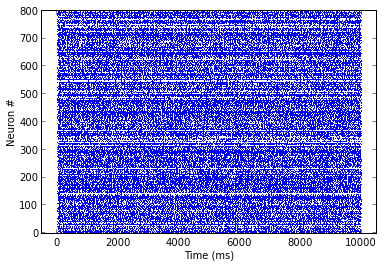In :
fig=subplot(131)
spikes.firing_rate(100, display=fig)
fig=subplot(132)
spikes.rate_distribution(display=fig)
fig=subplot(133)
spikes.cv_isi_hist(display=fig)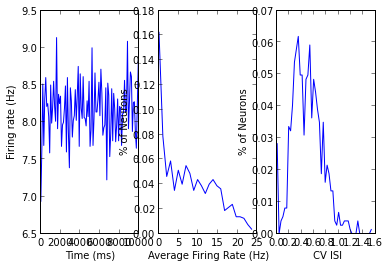As we can see, we have a constant firing rate at $\simeq$ 8.5Hz, with a ISI CV of 0.3, so pretty regular. The network is therefore in a SI state. To check that the boundaries conditions were not introducing any biases, we can also plot the average firing rate per neurons according to their positions:

In :
spikes.activity_map(float_positions=positions[:800, 1:3].T, display=True)